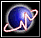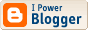Yet another weird SF fan
 I'm a mathematician, a libertarian, and a science-fiction fan. Common sense? What's that?Go to first entry

## Archives

<< current

 Yet another weird SF fan

### Another SF Cliche Bites the Dust

I'm referrring to nanowire. In Clicheland, monomolecular wire is supposed to be so thin, sharp, and tough that it will slice through flesh and bone with hardly any resistance. Any terrorist (or even an irresponsible twit) can loop it across a doorway and slice any passerby to ribbons. I doubt if it will work that way.

Let's make the following assumptions:

1. The wire is just strong enough to support an orbital tower;
2. it has about the same density as diamond;
3. it is 10 angstroms thick (an area of 100 square angstroms).

The strength-to-weight ratio needed to support an orbital tower is approximately the gravitational potential of the planet. In the case of Earth that would be about 6 × 107 newton-meters/kilogram. Diamond's density is about 3 × 103 kilograms/cubic meter. Put them together and we obtain a strength of about 2 × 1011 newtons/square meter (I'm keeping everything precise to only one decimal place). 100 square angstroms is 10−18 square meters so monomolecular wire can support 2 × 10−7 newtons. Not much.

Let's now consider what happens if it's looped across a doorway and someone walks through it. If the doorway is one meter wide and it starts cutting after it has been displaced one centimeter then it can exert a force of only 4 × 10−9 newtons. (If it starts cutting sooner then it can exert even less force.) If it is extended across one centimeter at that time, it will exert the force over an area of 10−11 square meters. That amounts to 400 newtons/square meter. Is this tolerable? Let's compare it to dental floss (people have cut themselves badly with dental floss so the comparison is not as preposterous as it might seem). If we assume that floss is about one millimeter wide and it extends one centimeter when looped around a finger then it has an area of 10−5 square meters. At 400 newtons/square meter, that's 4 × 10−3 newtons which is about one eightieth of an ounce. Since dental floss pressing on one's skin with a force of eightieth of an ounce is harmless, I think the dangers of monomolecular wire have been exaggerated.

The same material at the thickness of dental floss can be quite dangerous. Floss is about one million thicker than the hypothetical wire and the superfloss can exert one trillion times as much force (and one million times the pressure). That amounts to 3 × 103 newtons or 800 pounds (enough for floss to cut). I suspect that one tenth of a millimeter is the most dangerous size. It's thin enough to be effectively invisible and thick enough to cut.

In a society where extremely strong materials are commonplace, it may become necessary to wear clothing made of the same material.

 Profiles My Blogger ProfileX-treme Tracker The Atom Feed